Test: The Z-Transform

# Test: The Z-Transform

Test Description

## 20 Questions MCQ Test GATE Electrical Engineering (EE) 2023 Mock Test Series | Test: The Z-Transform

Test: The Z-Transform for Railways 2023 is part of GATE Electrical Engineering (EE) 2023 Mock Test Series preparation. The Test: The Z-Transform questions and answers have been prepared according to the Railways exam syllabus.The Test: The Z-Transform MCQs are made for Railways 2023 Exam. Find important definitions, questions, notes, meanings, examples, exercises, MCQs and online tests for Test: The Z-Transform below.
Solutions of Test: The Z-Transform questions in English are available as part of our GATE Electrical Engineering (EE) 2023 Mock Test Series for Railways & Test: The Z-Transform solutions in Hindi for GATE Electrical Engineering (EE) 2023 Mock Test Series course. Download more important topics, notes, lectures and mock test series for Railways Exam by signing up for free. Attempt Test: The Z-Transform | 20 questions in 60 minutes | Mock test for Railways preparation | Free important questions MCQ to study GATE Electrical Engineering (EE) 2023 Mock Test Series for Railways Exam | Download free PDF with solutions
 1 Crore+ students have signed up on EduRev. Have you?
Test: The Z-Transform - Question 1

###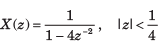​ ​ ​

Detailed Solution for Test: The Z-Transform - Question 1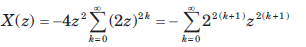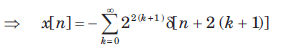Test: The Z-Transform - Question 2

### X(z) = ln(1 + z-1), |z| > 0

Detailed Solution for Test: The Z-Transform - Question 2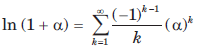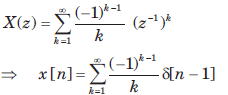Test: The Z-Transform - Question 3

### If z-transform is given by X(z) = cos(z-3), |z| > 0 The value of x is

Detailed Solution for Test: The Z-Transform - Question 3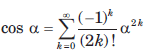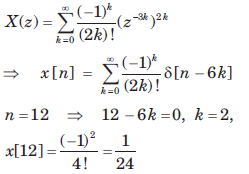Test: The Z-Transform - Question 4

X(z) of a system is specified by a pole zero pattern in fig.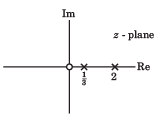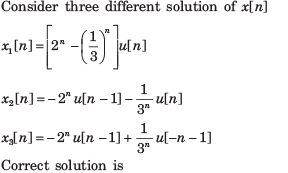Detailed Solution for Test: The Z-Transform - Question 4

All gives the same z transform with different ROC. So all are the solution.

Test: The Z-Transform - Question 5

Consider three different signal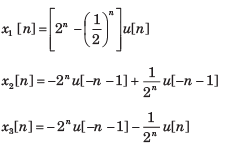fig.shows the three different region. Choose the correct option for the ROC of signal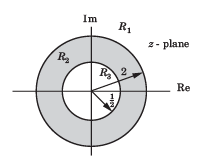R1  , R2 , R3

Detailed Solution for Test: The Z-Transform - Question 5

x1[n] is right-sided signal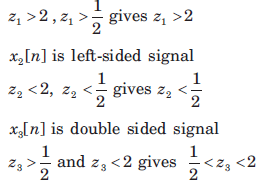Test: The Z-Transform - Question 6

Given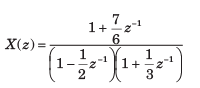For three different ROC consider there different solution of signal x[n] :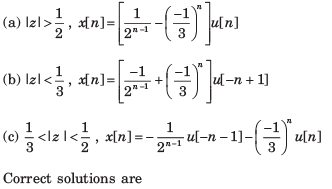Detailed Solution for Test: The Z-Transform - Question 6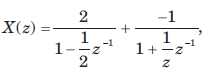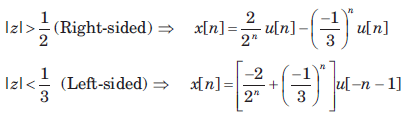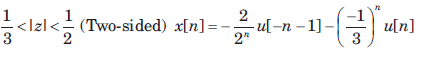Test: The Z-Transform - Question 7

X(z) has poles at z =1/2 and z =-1.If x  = 1 x [-1] = 1, and the ROC includes the point z = 34. The time signal x[n] is

Detailed Solution for Test: The Z-Transform - Question 7

Since the ROC includes the z = 3/4, ROC is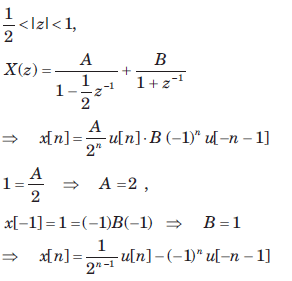Test: The Z-Transform - Question 8

x[n] is right-sided, X (z) has a signal pole, and x = 2, x = 1/2. x[n] is

Detailed Solution for Test: The Z-Transform - Question 8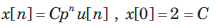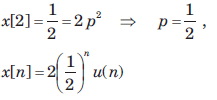Test: The Z-Transform - Question 9

The z-transform function of a stable system is given as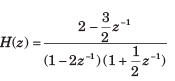The impuse response h[n] is

Detailed Solution for Test: The Z-Transform - Question 9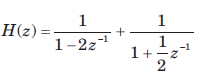h[n] is stable, so ROC includes |z| = 1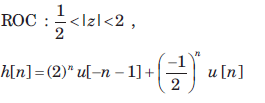Test: The Z-Transform - Question 10

Let x[n] = δ[n-2] + δ[n+2]  The unilateral z-transform is

Detailed Solution for Test: The Z-Transform - Question 10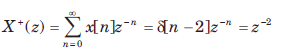Test: The Z-Transform - Question 11

The unilateral z-transform of signal x[n] = u[ n + 4 ] is

Detailed Solution for Test: The Z-Transform - Question 11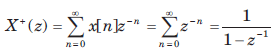Test: The Z-Transform - Question 12

The z-transform of a signal x[n] is given by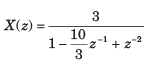If X (z) converges on the unit circle, x[n] is

Detailed Solution for Test: The Z-Transform - Question 12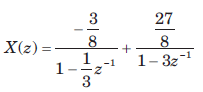Since X(z) converges on |z| = 1. So ROC must include this circle.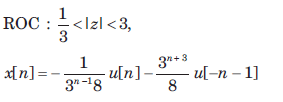Test: The Z-Transform - Question 13

The transfer function of a system is given as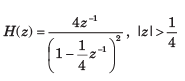The h[n] is

Detailed Solution for Test: The Z-Transform - Question 13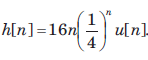So system is both stable and causal. ROC includes z = 1.

Test: The Z-Transform - Question 14

The transfer function of a system is given as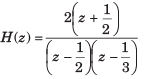Consider the two statements

Statement(i) : System is causal and stable.
Statement(ii) : Inverse system is causal and stable.

The correct option is

Detailed Solution for Test: The Z-Transform - Question 14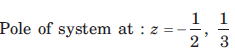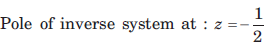For this system and inverse system all poles are inside |z| = 1. So both system are both causal and stable.

Test: The Z-Transform - Question 15

The impulse response of a system is given by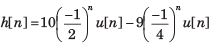For this system two statement are

Statement (i): System is causal and stable

Statement (ii): Inverse system is causal and stable.

The correct option is

Detailed Solution for Test: The Z-Transform - Question 15

Pole of this system are inside |z| = 1. So the system is stable and causal.
For the inverse system not all pole are inside |z| = 1. So inverse system is not stable and causal

Test: The Z-Transform - Question 16

The system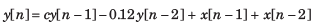is stable if

Detailed Solution for Test: The Z-Transform - Question 16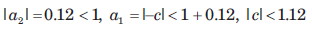Test: The Z-Transform - Question 17

Consider the following three systems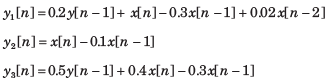The equivalent system are

Detailed Solution for Test: The Z-Transform - Question 17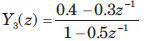So y1 and y2 are equivalent.

Test: The Z-Transform - Question 18

The z-transform of a causal system is given as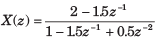The x is

Detailed Solution for Test: The Z-Transform - Question 18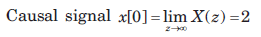Test: The Z-Transform - Question 19

The z-transform of a anti causal system is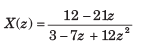The value of x is

Detailed Solution for Test: The Z-Transform - Question 19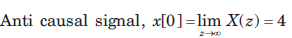Test: The Z-Transform - Question 20

Given the z-transforms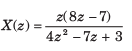The limit of x[ ∞] is

Detailed Solution for Test: The Z-Transform - Question 20

The function has poles at z = 1,3/4. Thus final value theorem applies.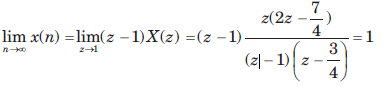## GATE Electrical Engineering (EE) 2023 Mock Test Series

22 docs|274 tests
Information about Test: The Z-Transform Page
In this test you can find the Exam questions for Test: The Z-Transform solved & explained in the simplest way possible. Besides giving Questions and answers for Test: The Z-Transform, EduRev gives you an ample number of Online tests for practice

## GATE Electrical Engineering (EE) 2023 Mock Test Series

22 docs|274 tests(Scan QR code)